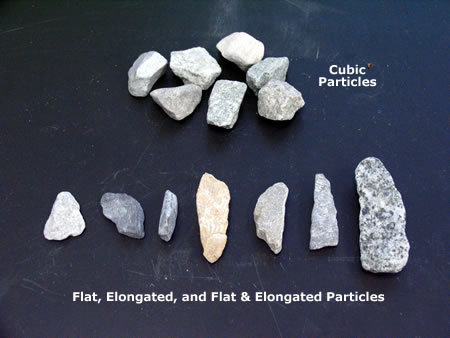# Aggregate Classification [Based on Shape]

According to shape the aggregate is classified as

• Rounded aggregate
• Irregular or partly rounded aggregate
• Angular aggregate
• Flaky aggregate
• Elongated aggregate
• Flaky and elongated aggregateAggregates of different shape

## 1. Rounded Aggregate

The aggregate with rounded shape has the minimum percentage of voids ranging from 32 to 33%. It gives minimum ratio of surface area to given volume and hence requires minimum water for lubrication. It gives good workability for the given amount of water and hence needs less cement for a given water cement ratio. The only disadvantages is that the interlocking between its particles is less and hence the development of bond is poor. This is why rounded aggregate is not suitable for high strength concrete and for pavements subjected to tension.

## 2. Irregular or Partly Rounded Aggregate

The aggregate with irregular shape has higher percentage of voids ranging from 35 to 37%. It gives lesser workability than rounded aggregate for the given water content. Water requirement is higher and hence more cement is needed for constant water cement ratio. The interlocking between aggregate particles is better than rounded aggregate but not adequate to be used for high strength concrete and pavements subjected to tension.

## 3. Angular Aggregate

The aggregate with angular shape has the maximum percentage of void ranging from 38 to 45%. It requires more water for lubrication and hence it gives least workability for the given water cement ratio. For constant water cement ratio and workability the requirement of cement increase. The interlocking between the aggregate particles is the best and hence the development of bond is very good. This is why angular aggregate is very suitable for high strength concrete and for pavements subjected to tension.

## 4. Flaky Aggregate

The aggregate is said to be flaky when its least dimension is less than 3/5th (or 60%) of its mean dimension. Mean dimension is the average size through which th particles pass and the sieve size on which these are retained. For example, mean size of the particles passing through 25 mm sieve and retained on 20 mm sieve is (20+25)/2=22.5 mm. if the least dimension is less than 3/5 x (22.5) = 13.5 mm, then the material is classified as flaky. Flaky aggregate tends to be oriented in one plane which affects the durability.

## 5. Elongated Aggregate

The aggregate is said to be elongated when its length is greater than 180% of its mean dimension.

## 6. Flaky & Elongated Aggregate

Aggregate is said to be flaky and elongated when it satisfies both the above conditions. Generally elongated or flaky particles in excess of 10 to 15% are not desirable.

Also Read: How to Classify Aggregate According to Size

6 Comments
1.2.3.4.•5.